Courses

# Test: Basics Part - 1

## 10 Questions MCQ Test Signal and System | Test: Basics Part - 1

Description
This mock test of Test: Basics Part - 1 for Electrical Engineering (EE) helps you for every Electrical Engineering (EE) entrance exam. This contains 10 Multiple Choice Questions for Electrical Engineering (EE) Test: Basics Part - 1 (mcq) to study with solutions a complete question bank. The solved questions answers in this Test: Basics Part - 1 quiz give you a good mix of easy questions and tough questions. Electrical Engineering (EE) students definitely take this Test: Basics Part - 1 exercise for a better result in the exam. You can find other Test: Basics Part - 1 extra questions, long questions & short questions for Electrical Engineering (EE) on EduRev as well by searching above.
QUESTION: 1

###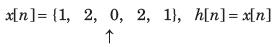Solution:

y[n] = {1,4, 4, 4, 10, 4, 4, 4, 1}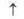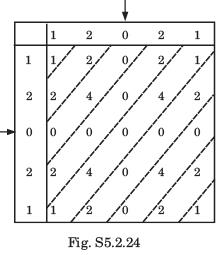QUESTION: 2

###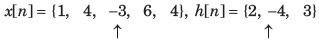Solution: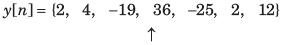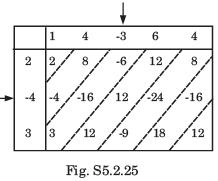QUESTION: 3

###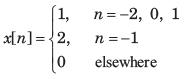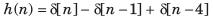Solution: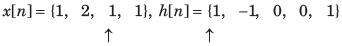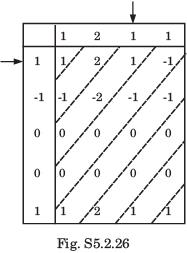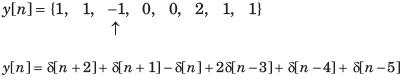QUESTION: 4

In question y[n] is the convolution of two signal. Choose correct option for y[n].
Q. y[n] = (-1)n * 2nu[2n+2]

Solution: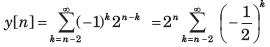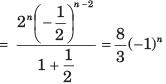QUESTION: 5

In question y[n] is the convolution of two signal. Choose correct option for y[n].
Q. y[n] =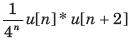Solution:

For n + 2<0 or n < -2, y [n] = 0
for n + 2 ≥ 0
or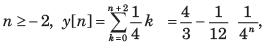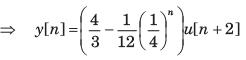QUESTION: 6

In question y[n] is the convolution of two signal. Choose correct option for y[n].
Q. y[n] = 3n u [-n + 3]* u[n-2]

Solution: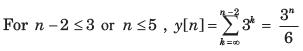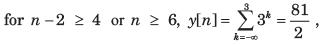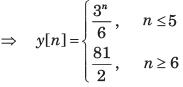QUESTION: 7

In question y[n] is the convolution of two signal. Choose correct option for y[n].
Q. y[n] = u [n + 3]* u[n-3]

Solution:

For n - 3 < -3 or n < 0, y[n] = 0
for n - 3≥ -3
or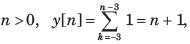y[n] = ( n + 1) u[ n]

QUESTION: 8

The convolution of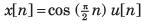and h[n] = u[n - 1] is f [ n]u[n - 1]. The function f [n] is

Solution:

For n - 1<0 or n < 1 , y[n] = 0
For n - 1 ≥ 0
or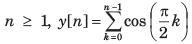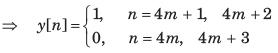QUESTION: 9

Let P be linearity, Q be time invariance, R be causality and S be stability. In question discrete time input x[n] and output y[n] relationship has been given. In the option properties of system has been given. Choose the option which match the properties for system.
Q. y[ n] = rect ( x[ n])

Solution: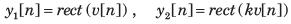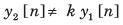(Not Homogeneous not linear)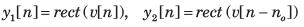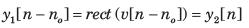(Time Invariant) At any discrete time n  = no , the response depends only on the excitation at that discrete time.
(Causal) No matter what values the excitation may have the response can only have the values zero or one.

QUESTION: 10

Let P be linearity, Q be time invariance, R be causality and S be stability. In question discrete time input x[n] and output y[n] relationship has been given. In the option properties of system has been given. Choose the option which match the properties for system.
Q. y[n] = nx[n]

Solution: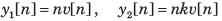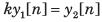(Homogeneous)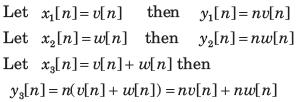= y1[n] + y2 [n] (Additive)
Since the system is homogeneous and additive, it is also linear.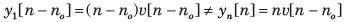(Time variant)
At any discrete time, n = no the response depends only on the excitation at that same time. (Causal)
If the excitation is a constant, the response is unbounded as n approaches infinity. (Unstable)